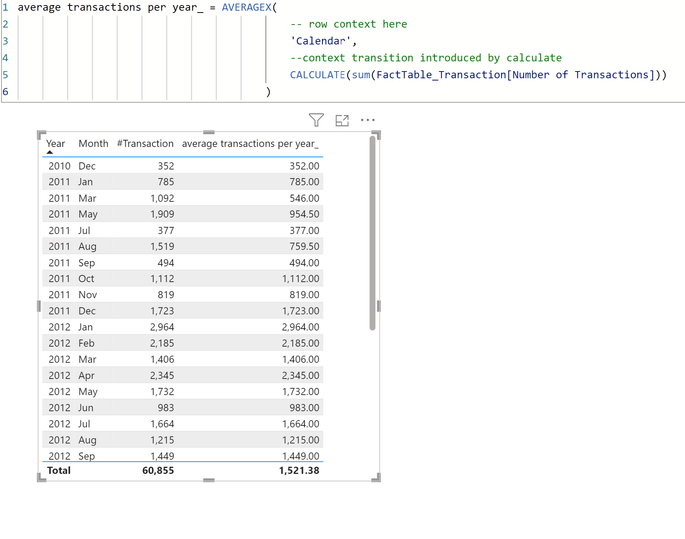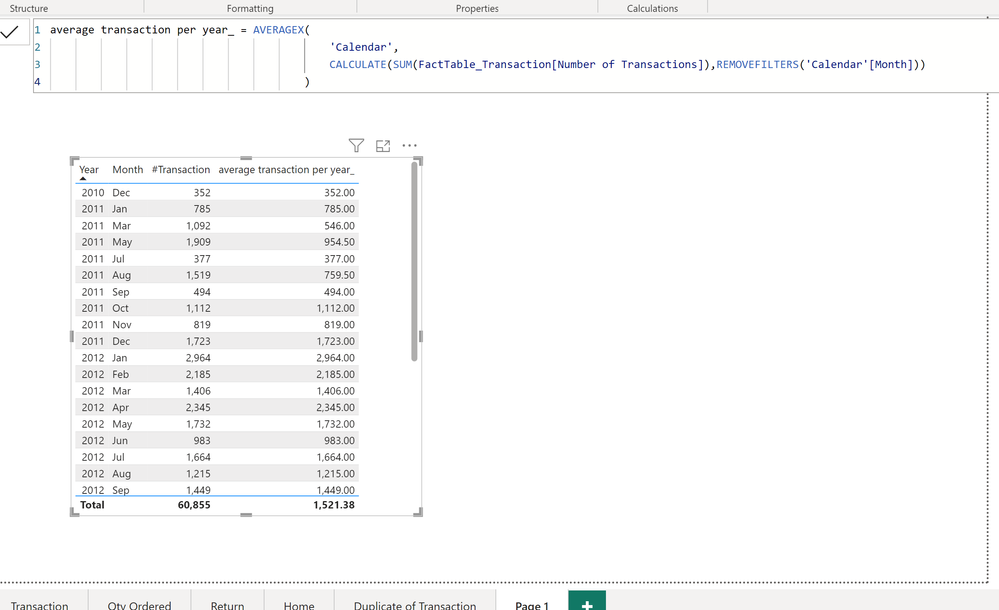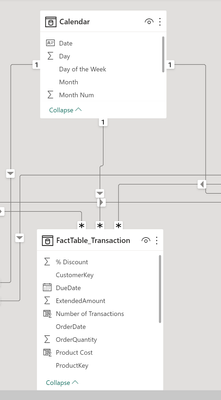cancel
Showing results for
Did you mean:

Fabric is Generally Available. Browse Fabric Presentations. Work towards your Fabric certification with the Cloud Skills Challenge.Frequent Visitor

## DAX REMOVEFILTER - not working as I expected

Hello,

I am trying to calculate the average transactions per year.
The Year and Month fields come from the Calendar table.

This is what I have so far:As I want to calculate the average transactions in each year, I add REMOVEFILTER('Calendar'[Month]) inside my CALCULATE function.The question is, why doesn't it have any effect? It still yields the same result as that without the REMOVEFILTERS.

This is my thinking, when you look at the visual say for March 2011, then we have 2 filters contexts to begin with: 'Calender'[Month]=March and 'Calendar'[Year]=2011. So when AVERAGEX iterates over the Calendar table, it filters this table for March and for 2011.Now since the CALCULATE function has REMOVEFILTERS('Calendar'[Month]) the filter 'Calendar'[Month]=March will be removed, so effectively we will be left with only just one filter and that is 'Calendar'[Year]=2011. So our Calendar table will be passing rows where Year =2011 to the CALCULATE function which will then filter the FactTable_Transaction for records where the date field is in 2011 (OrderDate field in FactTable_Transactions). This is context transtion.

Here is the excel file representing the FactTable_Transactions filtered for OrderDate in 2011.
As you can see from the snapshot a quick count shows 9830 records.

So there were 9830 transactions in 2011.In 2011, there were 12 distinct dates.
Here is the snapshot for this.So the AVERAGEX function will perform this calculation 9830/12 = 819.16

So in my visual I was expecting to see 819.16 eveywhere for when Year = 2011 irrrespective of the month.

This is my data model showing the relationship between Calendar and FactTable_Transaction. Its a 1-to-many relationship.Thank You

15 REPLIES 15Super User

That is too much read 😅

However, tye reason for such behavior is most probably the sort by column. If you extract the query code and paste it in DAX Studio, you'll find that the sort by column is part of the SUMMARIZECOLUMNS query, therefore it's part of the filter context.
Try the sam dax by this time using ALL ( 'Calendar'[Month], 'Calendar'[Month Num] )Super User

Hi @tamerj1 ,

What @mp390988 wants to find out is why his initial measure, even when adding the sort by column in the REMOVEFILTERS, is still not working for him:

``````Avg transactions by year wrong =
AVERAGEX(
'Date',
CALCULATE(
SUM('Table'[Qty]),
REMOVEFILTERS('Date'[Month],'Date'[Month Number])
)
)``````

While the suggested measure is working:

``````Avg transactions by year =
CALCULATE(
AVERAGEX(
'Date',
CALCULATE(SUM('Table'[Qty]))
),
REMOVEFILTERS('Date'[Month],'Date'[Month Number])
)``````Regards,

If this post answered your question, please mark it as a solution to help other users find useful content.
Kudos are another nice way to acknowledge those who tried to help you.

J. Payeras
Mallorca, SpainSuper User

That is clear. The measure iterates over the complete date table which creates a new filter context that includes all the columns of the date table. In this case in order for tge inner calculate to be able to remove the filter from the date table we need to remove all the filter from all the columns except year either using ALLEXCEPT or ALL ( 'Date' ) + VALUES ( 'Date'[Year] ) or ALLSELECTED ( 'Date' ) + VALUES ( 'Date'[Year] )

The 2nd measure the filter removal is done in the outer CALCULATE (outside the iteration over 'Date') thus is effective.Frequent Visitor

@tamerj1  -  can you please provide the DAX in order for my inner calculate to remove the filter from date table?Super User

Why? You already have a workable solution. Why do you have to do it in the inner CALCULATE? Do you have a practical reason for that or just for deep learning of how CALCULATE and context transition works?Frequent Visitor

Hi @tamerj1 ,

Yes, I have a workable solution.
But the fact that I initially tried to do it via the inner calculate and it did not give the desired results means there is some gaps in my knowledge/understanding which is why I am keen to know how to do it properly via the inner calculate. Basically, it is just for deep learning.

Anyways, you mentioned I need to remove the filter from the date table in the inner calculate using either:

1. ALLEXCEPT
2. ALL ( 'Date' ) + VALUES ( 'Date'[Year] )
3. ALLSELECTED ( 'Date' ) + VALUES ( 'Date'[Year] )

I tried with ALLEXCEPT as follows but this doesn't calcualte the average, instead it just gives the total for each year which I am not sure why.``````Average Transactions Per Year = AVERAGEX(
SUMMARIZE('Calendar','Calendar'[Month]),
CALCULATE(SUM(FactTable_Transaction[Number of Transactions]),ALLEXCEPT('Calendar','Calendar'[Year])
))``````Super User

You are right. In general try as much to avoid using CALCULATE approach unless it really simplifies the solution or improves the performance. Using CALCULATE approach requires a following a check list to avoid any mistake.

the 'Date' table that is being iterated by AVERAGEX is in fact calculated in the current filter context. Therefore it contains only the dates of the month available in the current filter context. Therefore no matter how you manipulate the inner CALCULATE filters, tge table that is being iterated will remain the same unless an outer CALCULATE is imposed. Or otherwise, the table needs to be calculated (removing the month filter) before being iterated.
On the other hand the inner CALCULATE provides context transition with creates a new filter context which replaces the outer filter context with a new one that contains all the columns from the date table. I guess you can imagine the complexity of the situation when using CALCULATE in such scenarios.
Many probable solutions are available to handle this case, one of them is your outer CALCULATE approach. You may also try

Avg transactions by year new =
AVERAGEX (
CALCULATETABLE ( 'Date', REMOVEFILTERS ( 'Date'[Month], 'Date'[Month Number] ) ),
CALCULATE ( SUM ( 'Table'[Qty] ) )
)Super User

Hi @mp390988 ,
I recommend to read through the Calculate evaluation steps:

CALCULATE – DAX Guide

I don't think I can explain it better but here are my two cents:

Why your REMOVEFILTERS('Calendar'[Month]) has not effect is because of Context Transition transforming the existing row context (while iterating your Calendar table) into an equivalent filter context.

See below an example of Calendar table:If you remove filters, while iterating, from 'Calendar'[Month] and 'Calendar'[Month Number] you still have the rest of filters active that's why you don't have any effect in your calculation.

I believe you want to call your Avg measure in a new filter context where the filter on Month and Month Number (coming from your visual are removed) and not removing those filters while iterating your Calendar table, I hope this makes sense to you.

``````Avg =
AVERAGEX(
'Date',
CALCULATE(SUM('Table'[Qty]))
)``````
``````Average transactions per year =
CALCULATE(
[Avg],
REMOVEFILTERS('Date'[Month],'Date'[Month Number])
)``````

Regards,

If this post answered your question, please mark it as a solution to help other users find useful content.
Kudos are another nice way to acknowledge those who tried to help you.

J. Payeras
Mallorca, SpainSuper User

Your visual looks like you applied a sort by column to the month. If you apply a sort by column, you need to remove fiters from the column shown and from the sort by column, like

...,

REMOVEFILTERS('Calendar'[Month], 'Calendar'[Sort Month])

...Super User

As I see it:

You could use REMOVEFILTERS instead of ALLEXCEPT, if you wish, but in the outer calculate not while iterating the Calendar table which does not remove the Month filter coming from the visual.Hope it helps.

If this post answered your question, please mark it as a solution to help other users find useful content.
Kudos are another nice way to acknowledge those who tried to help you.

J. Payeras
Mallorca, SpainFrequent Visitor

I believe this is the key for me to understand:

but in the outer calculate not while iterating the Calendar table

why is this?Frequent Visitor
Ok but just for my understanding why does adding REMOVEFILTER have no effect? i.e. why is it giving the same result as my DAX that doesn't contain REMOVEFILTER.

DAX with REMOVEFILTERS
average transactions per year_ = AVERAGEX(
'Calendar',
CALCULATE(SUM(FactTable_Transaction[Number of Transactions]),REMOVEFILTERS('Calendar'[Month]))
)

DAX WITHOUT REMOVEFILTERS:
average transactions per year_ = AVERAGEX(
'Calendar',
CALCULATE(SUM(FactTable_Transaction[Number of Transactions])
)

They both give same result and I am confused why this is happening as I imagined the REMOVEFILTERS to remove the filters from the monthSuper User

Hi again @mp390988 ,

I see what you mean...

See below an example where it works (3 months with a total Qty of 5 => 1,67).

The ALLEXCEPT goes with a CALCULATE wrapping the AVERAGEX.Hope this helps.

If this post answered your question, please mark it as a solution to help other users find useful content.
Kudos are another nice way to acknowledge those who tried to help you.

J. Payeras
Mallorca, SpainFrequent Visitor

Hi @Payeras_BI ,

Unfortunately, that gives the total transactions for year not the average.
I am looking for the average.ThanksSuper User

Hi @mp390988 ,

Try with ALLEXCEPT('Calendar','Calendar'[Year]) instead of REMOVEFILTERS('Calendar'[Month]).

If this post answered your question, please mark it as a solution to help other users find useful content.
Kudos are another nice way to acknowledge those who tried to help you.

J. Payeras
Mallorca, SpainAnnouncements#### Power BI Monthly Update - November 2023

Check out the November 2023 Power BI update to learn about new features.#### Fabric Community News unified experience

Read the latest Fabric Community announcements, including updates on Power BI, Synapse, Data Factory and Data Activator.#### The largest Power BI and Fabric virtual conference

130+ sessions, 130+ speakers, Product managers, MVPs, and experts. All about Power BI and Fabric. Attend online or watch the recordings.Top Solution Authors
Top Kudoed Authors
Users online (4,307)